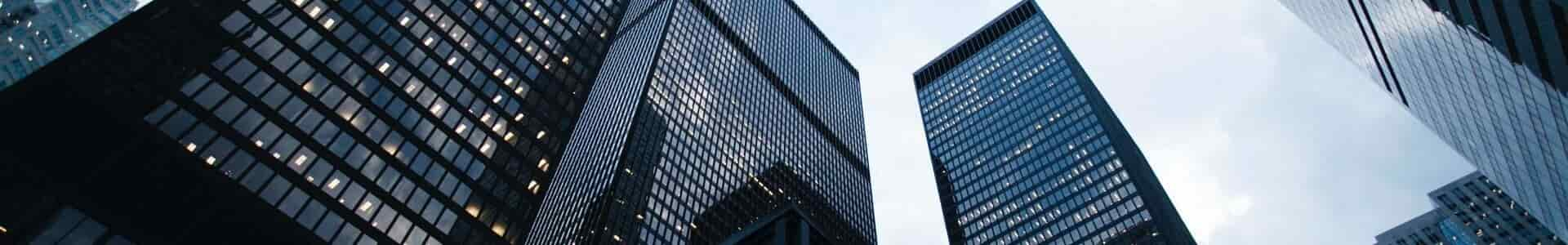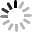NEWS
Home > news > Company news about What are the basic characteristics of stepping motor?
EVENTS
86-020-36907671
Contact Now

# What are the basic characteristics of stepping motor?

2023-02-13The following figure shows the relationship between torque and speed of stepping motor. The longitudinal axis is torque, and the transverse axis is pulse frequency. Pulse frequency refers to the frequency of driving pulse. In stepper motors, pulse frequency pps (pulses per second) is usually used instead of frequency Hz. The blue curve represents the "pull-in torque characteristic" of the stepping motor, and the yellow curve represents the "out-of-step torque characteristic" of the stepping motor.Each feature is described in the following sections:

·Pull-in torque characteristics
"Traction torque characteristic", also known as "starting torque characteristic", refers to the relationship between the starting frequency (pulse frequency) of the stepper motor in the stopped state and the load torque. The area within the traction torque curve is called "self-starting area", which can be started, stopped and reversed. In addition, the frequency at which the load torque is zero=the limit frequency at which the stepping motor can be started is called the "maximum self-starting frequency". As shown in the figure, the higher the frequency, the lower the starting load torque.

·Pull-out torque characteristics
"Out-of-step torque characteristic" is also known as "continuous characteristic" or "pull-out torque characteristic". Indicates the frequency at which the rotation can continue when the load torque is increased after starting. Therefore, its value is higher than the value of the pull-in torque characteristic. The limit of continuous operation of stepping motor is called "maximum continuous operation frequency". Like the pull-in torque characteristic, the out-of-step torque characteristic is that the load torque will decrease with the increase of pulse frequency.

·Holding Torque
When the stepping motor is powered on, even if external force is applied when the stepping motor stops, the motor also tries to maintain the stop position through the attraction between the rotor and the stator. This holding force is called "holding torque". In the figure above, the working frequency (pulse frequency) is zero, which is the torque in the stop state.
By the way, the torque of the stepping motor decreases with the increase of the working frequency because the current is difficult to flow at high frequency due to the influence of the winding inductance.
In addition, the pull-in torque characteristics and out-of-step torque characteristics of the stepping motor will vary with the excitation method and drive circuit. Therefore, in the study of the characteristics of stepping motor, it is necessary to carry out the overall evaluation including the driving method and circuit.

Key points:

·"Traction torque characteristic", also known as "starting torque characteristic", refers to the relationship between the starting frequency (pulse frequency) of the stepper motor in the stopped state and the load torque.

·The area within the traction torque curve is called "self-starting area", which can be started, stopped and reversed.

·The frequency at which the load torque is zero=the limit frequency at which the stepping motor can be started is called the "maximum self-starting frequency".

·"Out-of-step torque characteristic", also known as "continuous characteristic" or "pull-in torque characteristic", refers to the frequency that can continue to rotate when the load torque is increased after starting, and its value is higher than the value of pull-in torque characteristic.

·The limit of continuous operation of stepping motor is called "maximum continuous operation frequency".

·Both pull-in torque characteristic and out-of-step torque characteristic are that the load torque will decrease with the increase of pulse frequency.

·Holding torque is the force that the stepping motor tries to maintain the stop position even if external force is applied when the stepping motor stops under the power-on state.

·The pull-in torque characteristics and out-of-step torque characteristics of stepping motor will vary with the excitation method and drive circuit.Send your inquiry directly to us